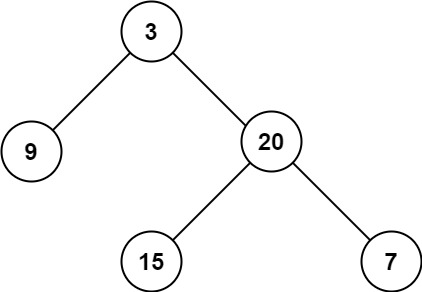# Minimum Depth of Binary Tree

## Problem Highlights

Understand what the interviewer is asking for by using test cases and questions about the problem.

• Established a set (2-3) of test cases to verify their own solution later.
• Established a set (1-2) of edge cases to verify their solution handles complexities.
• Have fully understood the problem and have no clarifying questions.
• Have you verified any Time/Space Constraints for this problem?
• What is the type of tree we are working with?
• The input tree will be a binary tree
• Can I assume the tree will be complete?
• No. A node may have less than 2 children
• Can I expect to receive an empty tree as input?
• Yes, the input tree can have 0-10000 nodes.
``````HAPPY CASE
Input: root = [3,9,20,null,null,15,7]
Output: 2````````````Input: root = [2,null,3,null,4,null,5,null,6]
Output: 5

EDGE CASE
Input: root = []
Output: 0``````

## 2: M-atch

Match what this problem looks like to known categories of problems, e.g. Linked List or Dynamic Programming, and strategies or patterns in those categories.

If you are dealing with Binary Trees some common techniques you can employ to help you solve the problem:

• Think about appropriate Tree Traversal: Pre-Order, In-Order, Post-Order, Level-Order
• Since we need to get the height of the tree, we will need to traverse all nodes down the tree, we should recursively return depth of each node upward to parent. a Pre-Order traversal would be appropriate
• Store nodes within a HashMap to refer to later
• We don't need to access the nodes or their values after they have been processed, so this technique is not useful for this problem
• Using Binary Search to find an element
• We are not looking to find an element in this problem so this technique is not useful for this problem
• Applying a level-order traversal with a queue
• Since we need to find the height of the tree, we could get nodes by level. A level-order traversal using a queue would be appropriate ## 3: P-lan

Plan the solution with appropriate visualizations and pseudocode.

General Idea: Recursively ask for child height in tree and add one for parent. Run recursive function on nodes only to check leaves only.

``````1. Basecase is a Null Node, return 0
2. Recursively check the minimum height from a node
a.If both children check min between depths
b.If single child check depth of child
c.If leaf return it's height.``````

⚠️ Common Mistakes

• Choosing the wrong traversal type
• Try to walk through the problem by hand and see the order in which you are processing the nodes. This will clue you into the type of traversal necessary

## 4: I-mplement

Implement the code to solve the algorithm.

``````# Definition for a binary tree node.
# class TreeNode:
#     def __init__(self, val=0, left=None, right=None):
#         self.val = val
#         self.left = left
#         self.right = right
class Solution:
def minDepth(self, root: Optional[TreeNode]) -> int:
# Basecase is a Null Node, return 0
if not root:
return 0

# Recursively check the minimum height from a node
if root.left and root.right:
# If both children check min between depths
return min(self.minDepth(root.left), self.minDepth(root.right)) + 1
elif root.right:
# If single child check depth of child
return self.minDepth(root.right) + 1
elif root.left:
# If single child check depth of child
return self.minDepth(root.left) + 1
else:
# If leaf return it's height.
return 1``````

## 5: R-eview

Review the code by running specific example(s) and recording values (watchlist) of your code's variables along the way.

• Trace through your code with an input to check for the expected output
• Catch possible edge cases and off-by-one errors

## 6: E-valuate

Evaluate the performance of your algorithm and state any strong/weak or future potential work.

Assume `N` represents the number of nodes in binary tree and `H` represents the height of the binary tree.

• Time Complexity: `O(N)`, because we need to visit each node in the binary tree
• Space Complexity: `O(N)` because we need to account for unbalanced tree (space is used for the recursion stack). In the general case `O(logN)` for balanced tree.